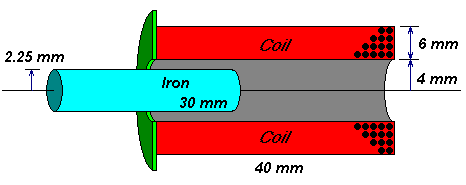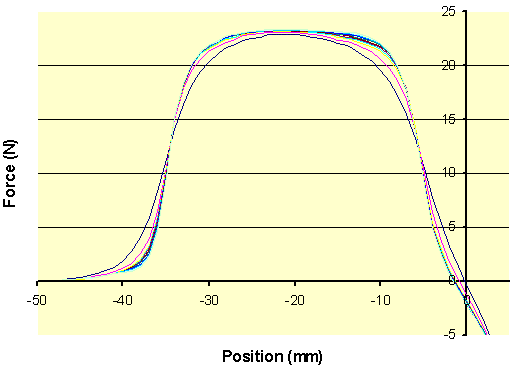# FEMM - Iron at Coil Entry

We've studied several air-core coils, isn't it about time we add iron? What thickness of iron works best? If you remember your early physics experiments, then you know a coil wound on a nail becomes many times stronger.

Note that we can't just add iron anywhere. For example, if you put the coil around an iron pipe, then all the magnetic flux goes into the pipe instead of the projectile. Not good. We need to put iron outside the coil somewhere.

We also want to study the whole range of motion. Some good work at the Magnetic Gun Club found the coil's force in the presence of magnetic shielding and end caps can increase the force by up to five times. But is this the whole story? It is possible that increased force at one point comes at the expense of decreased force elsewhere. So we'll use scripting to move the projectile through the entire range of influence, and find the total work done.

The simplest place to add iron is at the entry to the coil, at the entry. In theory it will shorten the path outside the coil that the flux must travel. It is easy to get a plain iron washer from the hardware store in a variety of sizes, and it sure doesn't get any simpler than that. But what thickness of iron will work best?

• Compute the projectile's kinetic energy, when the iron washer is of various thicknesses.
• The Lua script illustrates how to pass a value from the solver script to the visualization script.
• The mesh size is optimized for much faster computation without loss of accuracy.

## FEMM ModelThe simplest arrangement of iron is to add an end cap (an iron washer) at the entry to the coil. This simulation varies its thickness and computes total work done on the projectile.

This model approximates my actual parts, using nice round numbers:

• 40mm long coil
• 8mm inside diameter (4mm radius)
• 20mm outside diameter (10mm radius)
• 16 AWG wire (1.29mm diameter)
• An inductor simulation suggests this has 4 layers of 31 turns (51 microhenries, 71 milliohms)

The projectile is made of iron welding rod:

• 30mm long (make it 25% shorter than coil)
• M-19 steel (not sure if this is correct, but it matches my other FEMM models)
• "air gap" is actually a plastic firing tube (not shown)

Like my other FEMM models, this model has some important assumptions:

• frequency is d.c. (no eddy currents)
• velocity is zero (every point is simulated at steady-state with no motion)
• projectile is M-19 steel (nonlinear BH curve)
• steady coil current (not a capacitive-discharge system)
• no losses due to friction (don't we wish!)

## Pre-processor Lua Program

 iron_entry_pre.lua (download) ```outfile = "iron_entry_results.txt" handle=openfile(outfile,"a") write(handle, "\nInvestigating iron washer at entry to coil\n") write(handle, "Coil is 40mm long\n") write(handle, " 4mm inside radius\n") write(handle, " 10mm outside radius\n") write(handle, " 4 layers of 31 turns each\n") write(handle, "Projectile is 10mm long\n") write(handle, " 2.25mm radius\n") write(handle, " made of M-19 steel\n") write(handle, " starting 40mm away from center\n") closefile(handle) -- Save the model in temp file just to be safe save_femm_file("temp.fem") seteditmode("group") -- Amount the washer grows in thickness increment = 0.5 -- Outer loop runs once for each washer thickness for i=0,10 do thickness = 0.1 + (i * increment) -- Announce the current test condition handle = openfile(outfile,"a") write(handle, "Iron washer thickness = ", thickness, "\n") closefile(handle) -- Inner loop nudges projectile along the firing axis. -- It starts 40mm away from center and we do a few extra -- points past center in case the magnetic field is not centered. for n=1,47 do showmesh() analyse() -- Save current position for post-processor handle = openfile("tempfile","w") pos = n-41 write(handle, pos) closefile(handle) run_post("iron_entry_post.lua") -- move projectile selectgroup(1) move_translate(0,1) end -- move projectile back to start selectgroup(1) move_translate(0,-47) -- increase washer thickness selectgroup(3) move_translate(0,-increment) end messagebox("All done!") ```

## Post-processor Lua Program

 iron_entry_post.lua (download) ```outfile = "iron_entry_results.txt" -- read projectile's position at its centerpoint handle = openfile("tempfile","r") position = read(handle,"*n") closefile(handle) -- define contour clockwise around the 10mm-long projectile -- it needs to be ~2 mesh units outside the iron length = 10 -- length of projectile (mm) r = 2.25 -- radius of projectile (mm) mesh = 0.4 -- mesh size inside iron projectile top = position + length/2 + mesh*2 bot = position - length/2 - mesh*2 add_contour(0, top) -- upper left add_contour(r+mesh*2,top) -- upper right add_contour(r+mesh*2,bot) -- lower right add_contour(0, bot) -- lower left add_contour(0, top) -- and back to start -- STRESS TENSOR: compute mechanical force upon contour around projectile radial_force_real, radial_force_imag, axial_force_real, axial_force_imag = line_integral(3) -- LORENTZ FORCE: mechanical force on coil -- Note: We can't use the (simpler) Lorentz force calculation because the -- coil's force is unbalanced due to a single iron end cap. -- groupselectblock(2) -- lorentz_force = blockintegral(12) * (-1) handle=openfile(outfile,"a") write(handle, position, "\t", axial_force_real, "\n") closefile(handle) exitpost() ```

## Results

You can see the detailed Excel spreadsheet here. You can get the raw results file here as it was written by FEMM. For each washer thickness, it computes the force on the projectile at many positions and sums the result.This numerical solution integrates force as it acts over the distance the projectile moves, yielding "work". We ignore friction and losses, and assume the work is converted completely into kinetic energy. The graph shows the total work done for the given continuous coil current.

 Thickness (mm) Work  (Joules) 0.1 204.8 0.6 204.9 1.1 204.8 1.6 204.7 2.1 204.9 2.6 205.0 3.1 204.7 3.6 204.7 4.1 204.9 4.6 204.8 5.1 204.9

The highest and lowest amounts of work differ by 1.5%.

## Conclusion

Obviously, there is no dependence on work and washer thickness. The total kinetic energy is practically the same for every washer!The shape of the force graphs reveals the rest of the story. Although the peak force has increased, the tails have lower force. And the total "force over a distance" remains constant.

The analysis of the Magnetic Gun Club was accurate, but its results do not match my model. It found up to a 5x increase in force, where this coil shows no improvement. I need to figure out why they are different. I speculate that differences are caused by coil geometry or iron substance. More work is needed.

For the single washer studied there is zero benefit in exit velocity, regardless of washer thickness. I believe the iron shows no improvement because the flux path is dominated by air. Look again at the magnetic circuit equivalent model and observe the flux path includes both end caps, and down the tube and back around the outside. The total reluctance is completely dominated by air. It will require iron be added to the entire circuit to gain any advantage.

 < Previous Page 6 of 8 Next >